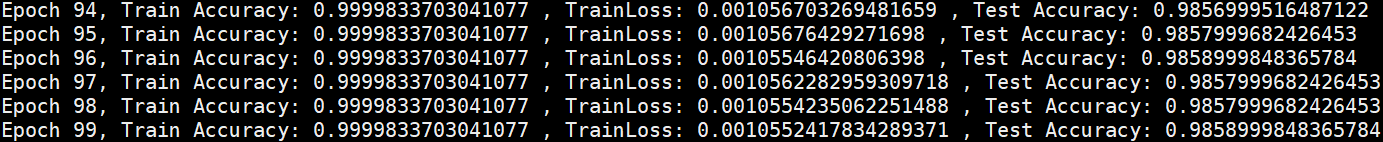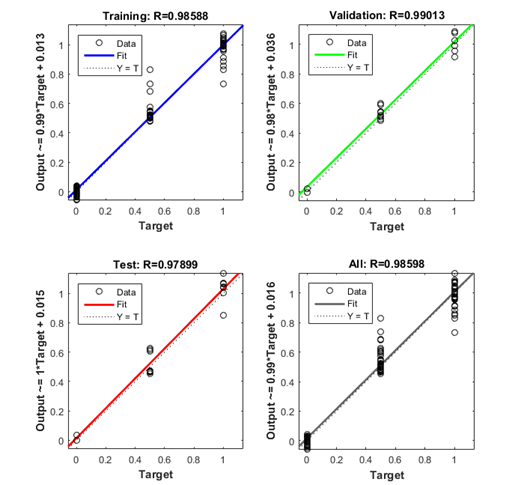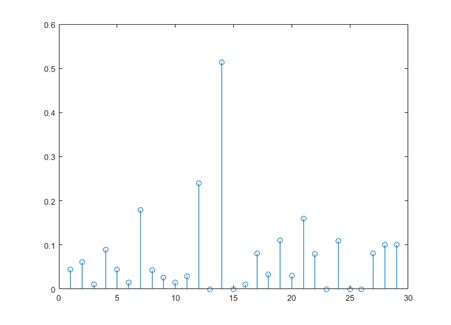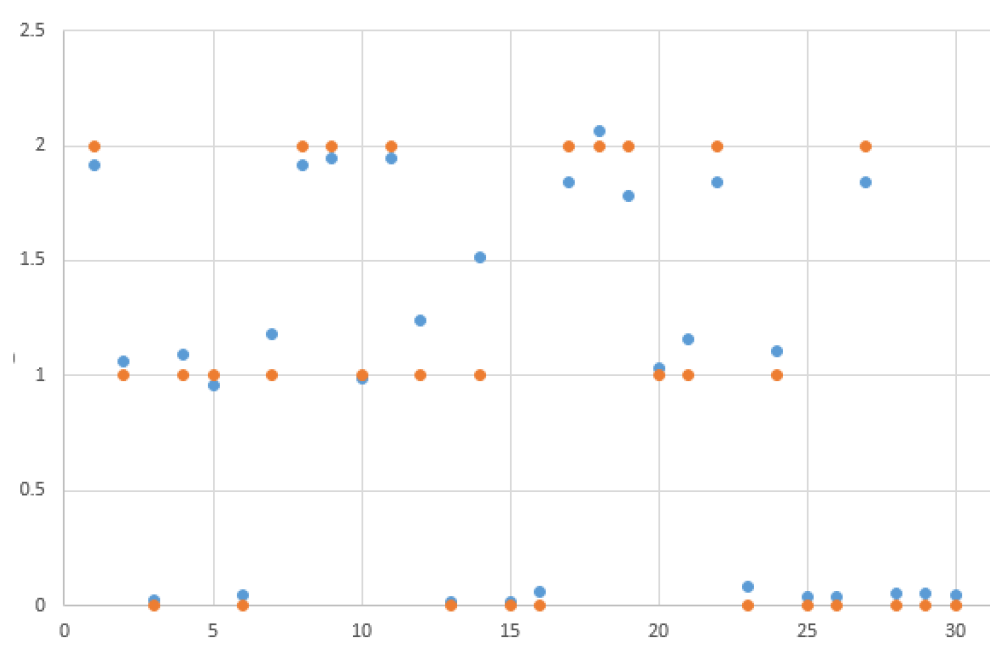• 神经网络是模拟人的思维过程对事件进行高校准确分类，分为学习过程和预测过程能够
• 针对多数机构面临的大规模报表数据录人问题，提出了一种基于BP神经网络的手写数字识别系统。对输人图像进行图像预处理、 图像分割和特征提取，随后将提取的特征信息输人到已经训练好的BP神经网络进行分类识别。
• 基于BP神经网络的遥感图像分类，王文文，郭嗣琮，为了研究神经网络在遥感分类中的应用，从神经网络的特点和其它的遥感分类方法的比较上，说明神经网络应用遥感分类有着独特的优势
• 基于深度学习的bP神经网络 进行图像分类 的代码实例bp神经网络
• 本文用Python实现了BP神经网络分类算法，根据鸢尾花的4个特征，实现3种鸢尾花的分类。 算法参考文章： iris_data_classification_bpnn_V1.py 需使用 bpnn_V1数据集 文件夹中的数据 iris_data_classification_bpnn_V2...
• 本代码主要是用BP神经网络进行数据分类，代码中是语音特征信号分类
•Python
• 设计一个BP神经网络对蝴蝶花进行分类 实验内容包括： BP神经网络的结构设计； 通过Python实现BP神经网络； 实验进行参数优化，对比不同参数的效果性能； 分析实验结果，优化BP神经网络结构；
• 训练集包括10种叶片，每种叶片20张图片，训练集数据为这200幅图片计算得到的叶片特征数据，每张图片提取10种形状特征参数，测试集数据和训练集数据一样，经过两层隐含层的bp神经网络训练，得到准确率和混淆矩阵图
• 基于BP神经网络的IRIS数据集训练和测试，有完整的数据集和实现代码，直接运行即可得到结果，并显示，正确率，误差，迭代次数等参数
• 文章以提取4类小麦碰撞声信号为基础，使用数字信号处理方法对小麦完好粒、虫害粒、霉变粒及发芽粒的碰撞声信号提取有效特征，最后利用BP神经网络进行分类，对于3类小麦类型的识别取得了较好的识别率。应用结果表明BP...
• 基于BP神经网络实现共享单车数据预测，环境：anaconda+jupyter notebook。文件包含代码+数据集
• 针对人体行为识别问题，提出一种基于径向基函数（BP）神经网络的人体行为分类算法。...最后，利用BP神经网络对样本进行分类；实验结果表明，与采用最近邻分类和K近邻分类（kNN）相比，所提算法具有更高的识别率。人体行为识别 SVD PCA LDA BP神经网络
• 本例中包含两层BP神经网络模板程序（可以直接调用，可定制中间层神经元个数，设置学习率，绘制衰减曲线，可用于简单的模式识别和预测）、一个调用的例程（包括简单的数据预处理如归一化的使用，测试结果准确率为98.3...
• 十分简单的BP神经网络程序，只有单层神经网络，适合初学者掌握TensorFlow。
• 基于BP神经网络的数据分类BP(BackPropagation)网络是1986年由Rumelhart和McCelland为首的科学家小组提出，是一种按误差逆传播算法训练的多层前馈网络，是目前应用最广泛的神经网络模型之一。BP网络能学习和存贮大量...

基于BP神经网络的数据分类

BP(Back

Propagation)网络是1986年由Rumelhart和McCelland为首的科学家小组提出，是一种按误差逆传播算法训练的多层前馈网络，是目前应用最广泛的神经网络模型之一。BP网络能学习和存贮大量的输入-输出模式映射关系，而无需事前揭示描述这种映射关系的数学方程。它的学习规则是使用最速下降法，通过反向传播来不断调整网络的权值和阈值，使网络的误差平方和最小。BP神经网络模型拓扑结构包括输入层(input)、隐层(hide layer)和输出层(output

layer)。

1 传统的BP算法简述

BP算法是一种有监督式的学习算法，其主要思想是：输入学习样本，使用反向传播算法对网络的权值和偏差进行反复的调整训练，使输出的向量与期望向量尽可能地接近，当网络输出层的误差平方和小于指定的误差时训练完成，保存网络的权值和偏差。具体步骤如下：

(1)初始化，随机给定各连接权及阀值。

(2)由给定的输入输出模式对计算隐层、输出层各单元输出

(3)计算新的连接权及阀值，计算公式如下：

(4)选取下一个输入模式对返回第2步反复训练直到网络设输出误差达到要求结束训练。

传统的BP算法，实质上是把一组样本输入/输出问题转化为一个非线性优化问题，并通过负梯度下降算法，利用迭代运算求解权值问题的一种学习方法，但其收敛速度慢且容易陷入局部极小，为此提出了一种新的算法，即高斯消元法。

2 改进的BP网络算法

2．1

改进算法概述

此前有人提出：任意选定一组自由权，通过对传递函数建立线性方程组，解得待求权。本文在此基础上将给定的目标输出直接作为线性方程等式代数和来建立线性方程组，不再通过对传递函数求逆来计算神经元的净输出，简化了运算步骤。没有采用误差反馈原理，因此用此法训练出来的神经网络结果与传统算法是等效的。其基本思想是：由所给的输入、输出模式对通过作用于神经网络来建立线性方程组，运用高斯消元法解线性方程组来求得未知权值，而未采用传统BP网络的非线性函数误差反馈寻优的思想。

2．2

改进算法的具体步骤

对给定的样本模式对，随机选定一组自由权，作为输出层和隐含层之间固定权值，通过传递函数计算隐层的实际输出，再将输出层与隐层间的权值作为待求量，直接将目标输出作为等式的右边建立方程组来求解。

(1)随机给定隐层和输入层间神经元的初始权值。

(2)由给定的样本输入计算出隐层的实际输出。

(3)计算输出层与隐层间的权值。以输出层的第r个神经元为对象，由给定的输出目标值作为等式的多项式值建立方程。

(4)重复第三步就可以求出输出层m个神经元的权值，以求的输出层的权矩阵加上随机固定的隐层与输入层的权值就等于神经网络最后训练的权矩阵。

3 计算机运算实例

%% 清空环境变量

clc

clear

%% 训练数据预测数据

data=importdata('test.txt');

%从1到768间随机排序

k=rand(1,768);

[m,n]=sort(k);

%输入输出数据

input=data(:,1:8);

output =data(:,9);

%随机提取500个样本为训练样本，268个样本为预测样本

input_train=input(n(1:500),:)';

output_train=output(n(1:500),:)';

input_test=input(n(501:768),:)';

output_test=output(n(501:768),:)';

%输入数据归一化

[inputn,inputps]=mapminmax(input_train);

%% BP网络训练

% %初始化网络结构

net=newff(inputn,output_train,10);

net.trainParam.epochs=1000;

net.trainParam.lr=0.1;

net.trainParam.goal=0.0000004;

%% 网络训练

net=train(net,inputn,output_train);

%% BP网络预测

%预测数据归一化

inputn_test=mapminmax('apply',input_test,inputps);

%网络预测输出

BPoutput=sim(net,inputn_test);

%% 结果分析

%根据网络输出找出数据属于哪类

BPoutput(find(BPoutput<0.5))=0;

BPoutput(find(BPoutput>=0.5))=1;

%% 结果分析

%画出预测种类和实际种类的分类图

figure(1)

plot(BPoutput,'og')

hold on

plot(output_test,'r*');

legend('预测类别','输出类别')

title('BP网络预测分类与实际类别比对','fontsize',12)

ylabel('类别标签','fontsize',12)

xlabel('样本数目','fontsize',12)

ylim([-0.5 1.5])

%预测正确率

rightnumber=0;

for i=1:size(output_test,2)

if

BPoutput(i)==output_test(i)

rightnumber=rightnumber+1;

end

end

rightratio=rightnumber/size(output_test,2)*100;

sprintf('测试准确率=%0.2f',rightratio)展开全文• 结合三个相关的程序和论文，一个是语音特征的分类（不调用神经网络工具箱相关函数实现），另外两个是关于手写数字识别的。处理的数据集是放在10个文件夹里，文件夹的名称对应存放的手写数字图片的数字，每个数字500...
• 包含两个图像分类的资源 1.bp算法 用matlab实现 图像...2.基于神经网络的图像分类，数据资源是基于CIFAR-10，内含训练测试数据（batch），是唐宇迪深度学习入门课程的修改代码，python3版本 源码公开，仅供学习使用。
• 一个基于BP神经网络的matlab程序，对几种字体0-9的数字识别
• 主要介绍了用Python实现BP神经网络（附代码），文中通过示例代码介绍的非常详细，对大家的学习或者工作具有一定的参考学习价值，需要的朋友们下面随着小编来一起学习学习吧
• 基于BP神经网络的遥感影像分类，俞冰，，传统统计模式识别方法进行遥感影像分类时要求数据服从正态分布，并且存在分类精度低的缺点。通过分析BP网的分类原理与学习算法，�
• 基于BP神经网络原理，针对数据当中所需的分类需求进行开发的小程序，具备一定的直到作用。
• 为提高垃圾识别与分类的效率，尤其是医疗废物，文中在图像识别的基础上，提出了基于BP神经网络的医疗废物识别与分类模型。该模型依据用户实时拍摄的图片，利用AGAST角点检测算法、FREAK描述算法、高阶局部自相关...
• 基于BP神经网络的图像分类

千次阅读 2020-04-16 22:40:20
本次BP神经网络分类实验所用数据集为MNIST手写数据集。 MNIST 数据集来自美国国家标准与技术研究所, National Institute of Standards and Technology (NIST)。训练集 (training set) 由来自 250 个不同人的手写的...

1 数据集

本次BP神经网络分类实验所用数据集为MNIST手写数据集。

MNIST 数据集来自美国国家标准与技术研究所, National Institute of Standards and Technology (NIST)。训练集 (training set) 由来自 250 个不同人的手写的数字构成, 其中 50% 是高中学生, 50% 来自人口普查局 (the Census Bureau) 的工作人员。测试集(test set) 也是同样比例的手写数字数据。

训练数据集包含60000个样本, 测试数据集包含10000样本。在 MNIST 数据集中，每张图片由28×28个像素点构成, 每个像素点用一个灰度值表示。使用时，将28×28的像素展开成一个维度为784的一维的向量, 向量中的值对应图片中的像素值。此外，还有一个标签数组，对应每张图片所属的手写数字类别（整数0-9）。

本次实验基于Pytorch框架，使用Pytorch自带工具包可完成MNIST数据集的下载及预处理。下图所示代码分别完成了训练数据和测试数据的下载，预处理及加载。

#1.Load the train datasets and test datasets
train_transformations = transforms.Compose([
transforms.ToTensor(),
transforms.Normalize((0.5,), (0.5,))
])
test_transformations = transforms.Compose([
transforms.ToTensor(),
transforms.Normalize((0.5,), (0.5,))
])

观察MNIST单个样本数据时，发现其中的像素值范围在(0,1)之间，说明已经被整除256，后续无需再重复这一操作。先将28×28的二维数据转换成张量Tensor的形式，再进行标准化处理，将数据映射到(-1,1)之间，最后将数据进行批处理，每批包含128（2的倍数，方便GPU处理）个样本，完成数据的加载。加载时设置打乱训练样本的顺序，不打乱测试样本的顺序。

2 分类网络设计

本次分类BP神经网络共有5层，包括1个输入层，3个隐藏层和一个输出层。层与层之间均采用全连接的形式。输入层有784个节点，对应MNIST单个样本的像素值总数；隐藏层的节点数分别为1024，1024，2048；输出层共有10个节点，用于分类，输出结果为（0-9）的预测概率。详细网络结构图如下所示。3 训练过程

训练过程主要分为以下四个步骤，分别为：

1. 加载训练数据集和测试数据集；

2. 初始化模型；

3. 定义优化器和损失函数；

4. 训练模型及保存权重参数；

下面对每个步骤详细展开介绍。

3.1 加载训练数据集和测试数据集

数据集的加载包括训练数据集和测试数据集的加载，训练数据集用于训练模型，测试数据集用于训练过程中对模型的性能进行实时监测。本次所用数据集为MNIST书写数字数据集，包括60000个训练样本和10000个测试样本，具体加载方式在第一章已详细介绍。

3.2 初始化模型

本次分类BP神经网络共有5层，包括1个输入层，3个隐藏层和一个输出层。层与层之间均采用全连接的形式。代码实现时，只需要实现3个隐藏层和1个输出层的设计即可。

每个隐藏层都由一个线性全连接层和一个激活函数构成，为了方便起见，自定义一个隐藏层模块，主要是定义线性全连接层的输入和输出节点数，激活函数的选取，实现过程如下所示。

# Build the neural network
class Unit(nn.Module):
def __init__(self, in_features, out_features):
super(Unit, self).__init__()

self.fc=nn.Linear(in_features, out_features, True)
self.relu = nn.ReLU()

def forward(self, input):
output = self.fc(input)
output = self.relu(output)
return output

接着，调用上面定义好的隐藏层模块实现整个网络的设计。将3个隐藏层根据其输入和输出节点数一一定义出来，再将这3个隐藏层组合成一个小网络。然后根据分类的类别数定义出输出层，也就是最后一个全连接层。最后在前向传播函数中，将这些层的输入输出拼接起来，实现整个网络的结构设计。代码实现如下所示。

class SimpleNet(nn.Module):
def __init__(self, num_classes=10):
super(SimpleNet, self).__init__()

self.unit1 = Unit(in_features=784,out_features=1024)
self.unit2 = Unit(in_features=1024, out_features=1024)
self.unit3 = Unit(in_features=1024, out_features=2048)
self.net = nn.Sequential(self.unit1, self.unit2, self.unit3)
self.fc = nn.Linear(in_features=2048,out_features=num_classes)

def forward(self, input):
output = self.net(input)
output = self.fc(output)
return output

完成网络结构设计后，通过实例化这个类来初始化训练模型。为了加速训练过程，选择在GPU上进行模型的训练和测试，具体过程如下所示。

#2.Initialize model
cuda_avail = torch.cuda.is_available()
model = SimpleNet(num_classes=10)
cuda_avail = torch.cuda.is_available()
if cuda_avail:
model.cuda()

3.3 定义优化器和损失函数

损失函数选用交叉熵损失函数，它是一种专门用于处理分类问题的损失函数。

#3. Define the optimizer and loss function
loss_fn = nn.CrossEntropyLoss()

3.4 训练模型及保存权重参数

定义训练过程，遍历整个训练集100轮。每遍历一轮训练集，根据训练轮次，不断调整学习率的大小，并计算模型在测试集上的准确率，若本轮训练后的测试准确率高于之前轮次，则保存本轮训练得到的权重参数。具体实现如下所示。

#4.Training
num_epochs = 100
best_acc = 0.0
for epoch in range(num_epochs):
train_acc = 0.0
train_loss = 0.0
optimizer,loss_fn,cuda_avail)
if test_acc > best_acc:
save_models(model,epoch)
best_acc = test_acc
print("Epoch {}, Train Accuracy: {} , TrainLoss: {} , Test Accuracy: {}".format(
epoch, train_acc, train_loss, test_acc))

训练过程如下所示。每一轮次训练结束均会打印训练精度，训练损失值和测试精度。4 完整代码

import torch
import torch.nn as nn
import os
from torchvision.datasets import MNIST
from torchvision.transforms import transforms
#Set GPU id
os.environ["CUDA_VISIBLE_DEVICES"] = "1"
# Build the neural network
class Unit(nn.Module):
def __init__(self, in_features, out_features):
super(Unit, self).__init__()

self.fc=nn.Linear(in_features, out_features, True)
self.relu = nn.ReLU()
def forward(self, input):
output = self.fc(input)
output = self.relu(output)
return output

class SimpleNet(nn.Module):
def __init__(self, num_classes=10):
super(SimpleNet, self).__init__()

self.unit1 = Unit(in_features=784,out_features=1024)
self.unit2 = Unit(in_features=1024, out_features=1024)
self.unit3 = Unit(in_features=1024, out_features=2048)
self.net = nn.Sequential(self.unit1, self.unit2, self.unit3)
self.fc = nn.Linear(in_features=2048,out_features=num_classes)

def forward(self, input):
output = self.net(input)
output = self.fc(output)
return output

# Create a learning rate adjustment function that divides the learning rate by 10 every 30 epochs
lr = 0.001
if epoch > 180:
lr = lr / 1000000
elif epoch > 150:
lr = lr / 100000
elif epoch > 120:
lr = lr / 10000
elif epoch > 90:
lr = lr / 1000
elif epoch > 60:
lr = lr / 100
elif epoch > 30:
lr = lr / 10
for param_group in optimizer.param_groups:
param_group["lr"] = lr

# Training process
model.train()
for i, (images, labels) in enumerate(train_loader):
if cuda_avail:
images = Variable(images.cuda())
images = images.reshape(-1,784)
labels = Variable(labels.cuda())
outputs = model(images)
loss = loss_fn(outputs, labels)
loss.backward()
optimizer.step()
train_loss += loss.cpu().data.numpy() * images.size(0)
_, prediction = torch.max(outputs.data, 1)
train_acc += torch.sum(prediction == labels.data)
train_acc = train_acc / 60000
train_loss = train_loss / 60000
return train_acc, train_loss

# Test the model while training
model.eval()
test_acc = 0.0
for i, (images, labels) in enumerate(test_loader):
if cuda_avail:
images = Variable(images.cuda())
images = images.reshape(-1,784)
labels = Variable(labels.cuda())

# Predict classes using images from the test set
outputs = model(images)
_, prediction = torch.max(outputs.data, 1)
test_acc += torch.sum(prediction == labels.data)

# Compute the average acc and loss over all 10000 test images
test_acc = test_acc / 10000
return test_acc

# Save the weights
def save_models(model,epoch):
torch.save(model.state_dict(), "MNIST_model_{}.model".format(epoch))
print("Chekcpoint saved")

def main():
#1.Load the train datasets and test datasets
train_transformations = transforms.Compose([
transforms.ToTensor(),
transforms.Normalize((0.5,), (0.5,))
])
test_transformations = transforms.Compose([
transforms.ToTensor(),
transforms.Normalize((0.5,), (0.5,))
])
#2.Initialize model
cuda_avail = torch.cuda.is_available()
model = SimpleNet(num_classes=10)
cuda_avail = torch.cuda.is_available()
if cuda_avail:
model.cuda()
#3. Define the optimizer and loss function
loss_fn = nn.CrossEntropyLoss()
#4.Training
num_epochs = 100
best_acc = 0.0
for epoch in range(num_epochs):
train_acc = 0.0
train_loss = 0.0
optimizer,loss_fn,cuda_avail)
if test_acc > best_acc:
save_models(model,epoch)
best_acc = test_acc
print("Epoch {}, Train Accuracy: {} , TrainLoss: {} , Test Accuracy: {}".format(
epoch, train_acc, train_loss, test_acc))

if __name__ == "__main__":
main()

展开全文神经网络 深度学习
• 随着我国葡萄酒业的逐步发展，葡萄酒生产企业的...其中将这178个样本的65%作为训练样本，另外35%作为测试样本，用训练样本对BP神经网络进行训练可以得到相对应的分类模型，在利用训练好的模型对测试样本进行分类识别。
• 参考周爱民教授机器学习公式推导过程及相关伪代码，使用简单易懂的语言将其编写出来，注释挺多，很适合编程新手。
• 基于BP神经网络的遥感影像分类方法研究，张建平，王崇倡，采用BP神经网络进行遥感影像分类，可以在一定程度上消除传统的遥感影像分类所带来的模糊性和不确定性。然而，BP网络自身也存在着�
• 基于MATLAB，BP神经网络用于二分类，以蚊子分类为例。内有实验代码，实验运行结果。matlab
• 基于BP神经网络的分类

千次阅读 2019-05-18 22:47:34
背景 使用的是一个简单的数据集fisheriris，该数据集数据类别分为3类，...其中meas是150*4的矩阵代表着有150个样本每个样本有4个属性描述，species代表着这150个样本的分类。 实验测试 原始的数据集中的标签...

背景

使用的是一个简单的数据集fisheriris，该数据集数据类别分为3类，setosa，versicolor，virginica。每类植物有50个样本，共150个样本代表150朵花瓣。每个样本有4个属性，分别为花萼长，花萼宽，花瓣长，花瓣宽。其中meas是150*4的矩阵代表着有150个样本每个样本有4个属性描述，species代表着这150个样本的分类。

实验测试

原始的数据集中的标签格式如下所示：

setosa

setosa

versicolor

versicolor

virginica

virginica

为了能够进行在BP神经网络训练时更好的进行处理，首先使用MATLAB将以上字符串标签更改为数字标签，其中setosa对应于数字1，versicolor对应于数字2，virginica对应于数字3。

然后使用MATLAB建立的神经网络结构，其中神经网络的输入值个数为3个，隐含层的个数为4层。神经网络训练参数的设置分别为：最大迭代次数1000次，训练的目标为10-3，学习率设置为0.01。

如图所示为使用MATLAB进行神经网络进行训练之后的回归结果，可以看出预测出的结果大致分成了三个部分，大都距离45度斜线很近。然后对训练后得到的30组结果进行反归一化后，再计算出的标签值与实际标签值的相对误差，画出30组测试集所得出的结果与测试集标签值相对误差的点线图如图所示。从图中我们可以看出，有一组数据结果的误差达到了50%以上，其余的误差大都在20%以下，由此结果是可以取得较高的准确率的。下图中为对应的计算出的标签值与实际标签值的对比，其中橘色的点为标签的世纪值，蓝色的点为使用BP神经网络计算出的标签值，可以看出在大多数情况下，每组数据的两个点之间的距离是比较接近的。本例中使用计算出的标签值与真实标签值相差30%以内视为预测正确，则图中所示预测正确率为96.67%。源码

clear all
clc

% 导入数据

%将标签从字符改变为数字
for i=1:150
temp1 = species(i,1);
if (strcmp(temp1,'setosa'))
label(i,1) = 0;
end
if (strcmp(temp1,'versicolor'))
label(i,1) = 1;
end
if (strcmp(temp1,'virginica'))
label(i,1) = 2;
end
end

% 随机产生训练集和测试集
temp = randperm(size(meas,1));
% 训练集——120个样本
P_train = meas(temp(1:120),:)';
T_train = label(temp(1:120),:)';
% 测试集——30个样本
P_test = meas(temp(121:end),:)';
T_test = label(temp(121:end),:)';
N = size(P_test,2);

% 数据归一化
[p_train, ps_input] = mapminmax(P_train,0,1);
p_test = mapminmax('apply',P_test,ps_input);

[t_train, ps_output] = mapminmax(T_train,0,1);

% 创建网络
net = newff(p_train,t_train,9);

%设置训练参数
net.trainParam.epochs = 1000;
net.trainParam.goal = 1e-3;
net.trainParam.lr = 0.01;

%训练网络
net = train(net,p_train,t_train);

%仿真测试
t_sim = sim(net,p_test);

% 数据反归一化
T_sim = mapminmax('reverse',t_sim,ps_output);

%相对误差error
error = abs(T_sim - T_test)./T_test;

%决定系数R^2
R2 = (N * sum(T_sim .* T_test) - sum(T_sim) * sum(T_test))^2 / ((N * sum((T_sim).^2) - (sum(T_sim))^2) * (N * sum((T_test).^2) - (sum(T_test))^2));

%结果对比
result = [T_test' T_sim' error']

%绘图
figure
plot(1:N,T_test,'b:*',1:N,T_sim,'r-o')
legend('真实标签值','预测结果')
xlabel('测试集')
ylabel('标签')
string = {'结果可视化对比';['R^2=' num2str(R2)]};
title(string)

j = 0;
for i=1:30
err=error(1,i)
if (err>0.3 && err~=Inf)
j=j+1;
end
end
rate = (30-j)/30

注：最后一个图是把数据粘贴出去使用Excel画的，所以以上程序执行出的第三个图会和博文中有差异 。

展开全文...